## Component Scaling in Mixture Designs¶

Three scales are used to transform the component levels to a convenient scale for analysis. The original scale used to input the component levels is the “Actual”. These are converted to the “Real” scale, with each component re-scaled so that the total is 1.0. In the real scale, the components are then fractions of each component in the mixture. If the total of the components is set to 80% then the “Real” values are the actual values divided by 0.80.

For analysis, the “Pseudo” scale is preferred. In the pseudo scale each component is rescaled to be a fraction of the “active” part of the mixture. L-Pseudo is the default scale, but U-Pseudo will be offered if collinearity is lower in this scale. (See mathematical description below.)

To illustrate, suppose that a three-component nut mixture will always contain at least 50% peanuts, at least 15% pecans and at least 5% cashews. Therefore, 70% of the nut mix is predetermined. We are going to do an experiment to determine the remaining 30%. In pseudo terms,

Peanut pseudo component = (% peanut – 50%) / 30%

Pecan pseudo component = (% pecan – 15%) / 30%

Cashew pseudo component = (% cashew – 5%) / 30%

If there are no lower limit constraints, the real and pseudo scales are identical.

After building the design you may change the scale via the Display Options menu.

### L-Pseudo vs U-Pseudo¶

Lower bounded pseudo values: the minimum value of each component becomes zero; the maximum value approaches one. How close it comes to one depends on the constraints. For a simplex the component minimums are zero and the maximums are one.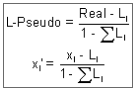Upper bounded pseudo values: the maximum value of each component becomes zero; the minimum value approaches one. How close it comes to one depends on the constraints. For an inverted simplex the component maximums are zero and the minimums are one.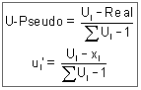## Slack Variable Model Form¶

In some cases of constrained mixtures, it becomes difficult to get a good regression fit of the Scheffe model. This occurs when you introduce a wide disparity in the constraint ranges. For example, you might make a blend with one ingredient, such as a catalyst, that’s very powerful, so you constrain it to fractions of volume percent, while all other ingredients can be varied over wide volumes. The resulting space will be a narrow “sliver” with very poor design properties. If it’s really bad, then Design-Expert will recommend you try the “slack variable” mixture model. This model resembles the usual RSM models except that one component must be removed, because it will be a linear combination of all the others. The best term to remove is usually the one with the widest range. You can change this if you like.

You cannot eliminate any of the other main effect terms, because they incorporate information on other terms. They will be forced into the model.

Because it excludes at least one component and all its interactions, we recommend that you not use the slack variable form if at all possible. Instead consider changing the metric of your mixture to a form that results in more even ranges. For example, you might convert from weight percent to mole percent in a chemical mixture. In any case, if it works, the design you get with the Scheffe model will likely be nearly as good as the slack form. You will still be able to analyze your data with the slack form should you feel it necessary.

We advise that you always evaluate your mixture design by using the Design Evaluation node before you run it. Any special problems with the design may then be discovered before it’s too late.

## Mixture Coding for Model Fitting¶

Design-Expert allows the user to specify the coding (pseudo, real or actual) to use for fitting the model.

• If you specify pseudo, the X-matrix uses pseudos for the coefficient

• calculations and the equation is back transformed to both reals and

• actuals.

• If you specify reals, the X-matrix uses reals for the coefficient

• calculations and the equation is back transformed to actuals, but not

• pseudos.

• If you specify actuals, the X-matrix uses actuals for the coefficient

• calculations and the equation is back transformed to reals, but not

• pseudos.

# Scheffé Mix Models¶

Scheffé models were specifically developed to handle the natural constraints of mixture designs.

Mixture models are only readily interpretable when the mixture components all go from 0 to the total for the design. Most mixture designs cover a more constrained space. Use the Model Graphs to better understand the models.

The Scheffé model forms are as follows:

## Linear¶

$\hat{y}= \sum_{i=1}^{q}\beta _{i} x_{i}$

### Example¶

$12A + 8B + 4C$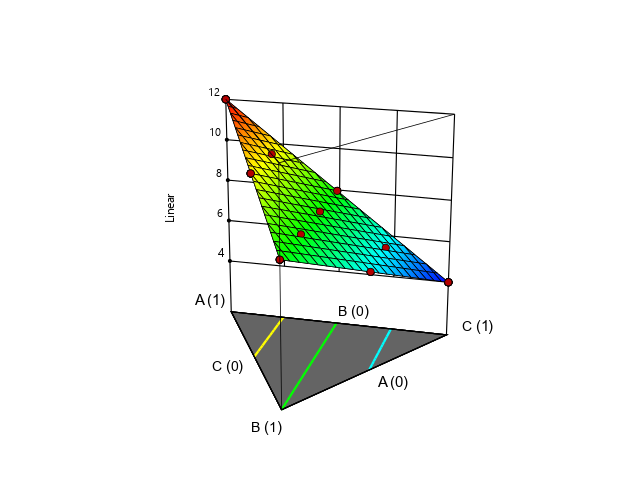Note that for a linear model the edges of the graphs are straight. In the unconstrained simplex the coefficient value is the prediction when the mixture is a pure component.

$\hat{y}=\sum_{i=1}^{q} \beta_{i}x_{i}\,+\sum_{i<j}^{q-1}\sum_{j}^{q} \beta_{ij} x_{i} x_{j}$

### Example¶

$12A + 8B +4C + 8AB - 8AC$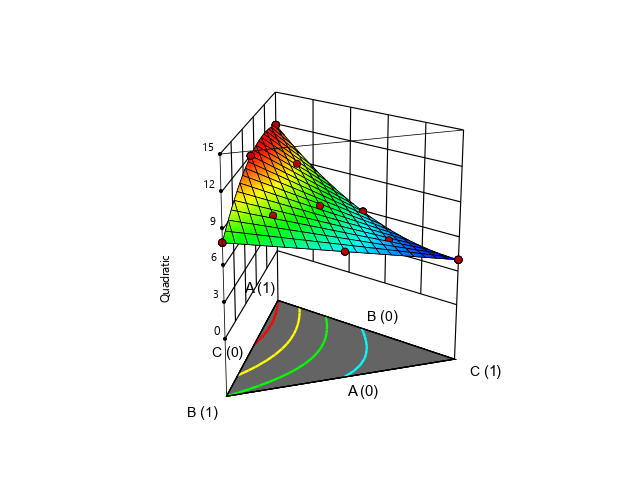When there are two component blending effects the edges curve away from the linear model by one-fourth of the coefficient at the 50/50% blend (1/2 squared).

## Special Cubic¶

$\hat{y} \sum_{i=1}^{q} \beta_{i}x_{i}+\sum_{i<j}^{q-1}\sum_{j}^{q} \beta_{ij}x_{i}x_{j}+\sum_{i<j}^{q-2}\sum_{j<k}^{q-1}\sum_{k}^{q}\beta_{ijk} x_{i}x_{j}x_{k}$

### Example¶

$12A + 8B + 4C + 8AC - 8BC + 54ABC$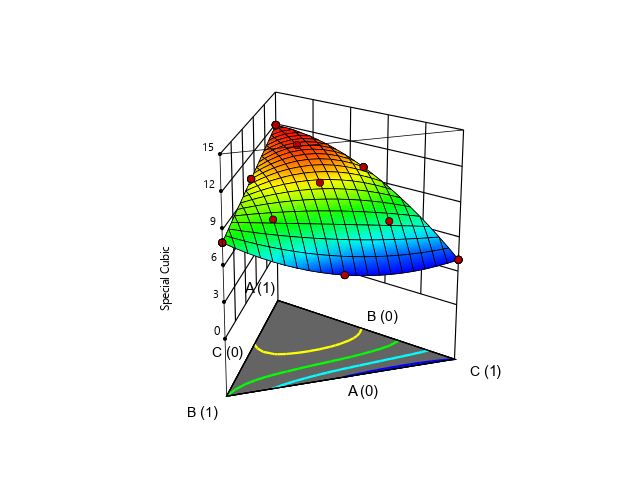When there is three component blending, the curve away happens in the middle of the simplex and is one-twenty-seventh (1/3 cubed) of the coefficient.

## Full Cubic¶

$\hat{y}=\sum_{i=1}^{q}\beta_{i}x_{i}+\sum_{i<j}^{q-1} \sum_{j}^{q}\beta_{ij}x_{i}x_{j}+\sum_{i<j}^{q-1}\sum_{j}^{q}\delta_{ij}x_{i} x_{j}(x_{i}-x_{j})+\sum_{i<j}^{q-2}\sum_{j<k}^{q-1}\sum_{k}^{q}\beta_{ijk}x_{i} x_{j}x_{k}$

### Example¶

$12A + 8B + 4C + 8AB - 8AC + 54ABC + 48AC(A - C)$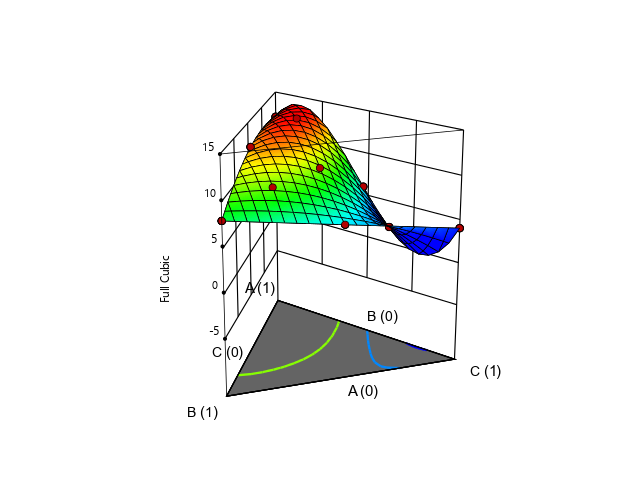Higher-order terms are used to model wavy surfaces.

Standard Scheffé polynomials are available up to the fourth order. There are also partial quadratic mixture (PQM) models using a combination of linear, squared, and quadratic terms.

References